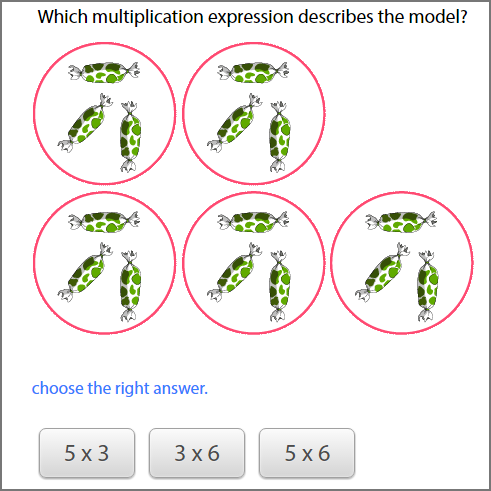# Identifying Multiplication Expression For Equal Groups - Recognise Equal Groups

## 'Identify multiplication expression for equal groups' Test and Printable Worksheets

Identifying multiplication sentence for equal groups is an excellent teaching tool for a perfect understanding of the multiplication concept.
In this 'identify multiplication expression for equal groups' test and printable worksheet, the essence is for kids to recognize equal groups and have a conceptual understanding of how multiplication can represent groups of the same size.

## Click the sections below to find more explanation on this topic

...

### Key to best performance - Identify multiplication expression for equal groups

Our test on identifying multiplication expression for equal groups offers the key to best performance.

Having obtained our printable worksheet, children can be able to see through the beautiful models, number of groups of objects and equal sizes.

This however makes it very easy for them to reason and identify multiplication expression for equal groups.
It is thus very essential for kids to experience the use of multiplication on a daily basis; such as grouping themselves for games, grouping food items to share amongst them, and so many others.

### The commutative property of multiplication expression

In multiplication, the commutative property states that order does not matter.

This insinuates that, the multiplication expression for example 2 x 4 is the same as 4 x 2.

Moreover, a thing worthy of note is that even if the order of factors change, or are reversed, the product still remains the same.

To give an illustration, 2 x 4 = 8 is same as 4 x 2 = 8.

### Example - Correct multiplication expression to describe model

Below are well designed models for you to identify their multiplication expressions.

Each model of equal groups, contain a series of multiplication expressions for you to choose the correct answer.

5 groups of sweets are presented on a model, with 3 sweets in each group.

Which among the following multiplication expression best describes the model?It is so simple. Isn't it? We've got 5 groups of sweets x 3 sweets in each group.

Thus our correct answer is 5 x 3

Hey sweet! It is very simple.